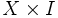# Cone space

Jump to: navigation, search
This article describes a construct that involves some variant of taking a product of a topological space with the unit interval and then making some identifications, typically at the endpoints, based on some specific maps.
View more such constructs

## Definition

Given a topological space$X$, the cone space of$X$, denoted as$CX$, is defined as the quotient of$X \times I$ (where$I$ is the closed unit interval$[0,1]$ and we use the product topology) by the equivalence relation:$(x_1,0) \sim (x_2,0) \forall x_1,x_2 \in X$

Here,$I$ refers to the closed unit interval$[0,1]$.

Refer: